# Aspect ratio

Determine the ratio of the length to the width of a picture frame that is 12 3/4 inches long and 8 1/2 inches wide.

r =  3:2

### Step-by-step explanation: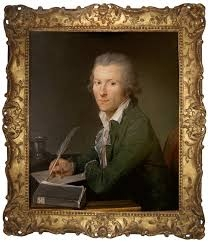Did you find an error or inaccuracy? Feel free to write us. Thank you!Tips to related online calculators
Need help to calculate sum, simplify or multiply fractions? Try our fraction calculator.
Check out our ratio calculator.

## Related math problems and questions:

• A box 2A box has a length of 4 1/2 inches, a width of 3 2/3 inches and a height of 8 1/4 inches. What is the volume of the box?
• Fractions and mixed numerals(a) Convert the following mixed numbers to improper fractions. i. 3 5/8 ii. 7 7/6 (b) Convert the following improper fraction to a mixed number. i. 13/4 ii. 78/5 (c) Simplify these fractions to their lowest terms. i. 36/42 ii. 27/45 2. evaluate the follow
• Frame image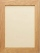Peter wants to put a picture in a frame. The picture is 7 3/5 cm wide. To fit in the picture frame cannot be more than 7 3/10 cm wide. How much should the image be trimmed?
• Long and wideWhat is the area of a rectangle that is 1 1/4 meters long and 3/4​ of a meter wide
• Evaluate mixed expressionsWhich of the following is equal to 4 and 2 over 3 divided by 3 and 1 over 2? A. 4 and 2 over 3 times 3 and 2 over 1 B. 14 over 3 times 2 over 7 C. 14 over 3 times 7 over 2 D. 42 over 3 times 2 over 31
• What is 9What is the value of x in the proportion 2 and one-fourth over x = 1 and one-half over 3 and three-fifths? 2 and two-fifths 5 and two-fifths 8 and 1 over 10 12 and 3 over 20
• Jewelry box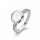The bottom of Zeyda's jewelry box is a rectangle with the length of 5 3/8 inches and a width of 3 1/4 inches. What is the area of the bottom of the jewelry box?
• Picture frames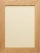Destiny has to make 24 picture frames. Each frame uses 16 3/8 wood trim. 2/3 were completed on the first day. How many inches of wood trim is needed to complete the remaining frames on the second day?
• Lin hasLin has a cake pan that has a length of 8/3 inches, a width of 15/4 inches and a height of 3/2 inches. What is the volume of the cake pan?
• Composite ratioJakub, Aneta, and Lenka divided 1342 USD in the ratio 5/2: 3/10: 1/4. How much did Lenka take?
• Thomas 2Thomas is 56 3/4 inches tall. Jillian is 1 3/8 inches shorter than Thomas and Jane is 1 1/5 inches shorter than Jillian. How tall is Jane? Label the names on the tape diagram to represent this problem. Jane Jillian Thomas Excellent!
• Bamboo 2A piece of bamboo is to 2 1/4 meter long. Jessie cuts 1 1/3 meter to be used for making some kites and another piece 3/4 meter long to be given to his friend. How long is the me remaining piece of bamboo?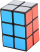A gift box has dimensions of: 8 1/2 inches, 5 1/2 inches, and 2 1/2 inches, respectively. How many cubes with side lengths of 1/2 inches would be needed to fill the gift box?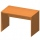Juila has a rectangular table that is 3 1/2 feet long and 1 3/4 feet wide. What is the area of Juila's table?Susanna is cutting construction paper into rectangles for a project. She needs to cut one rectangle that is 10 inches × 14 1/2 inches. She needs to cut another rectangle that is 10 1/3 inches by 10 1/2 inches. How many total square inches of constructionRose spends 2 1/3 hours studying Math, 1 3/4 hours studying English, and 2 1/4 hours studying Science. Find her average time studying the three subjects.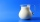Carlos mixed 4/15 of chocolate syrup with 1/2 of milk. Determine the reasonable estimate of the total amount of liquid